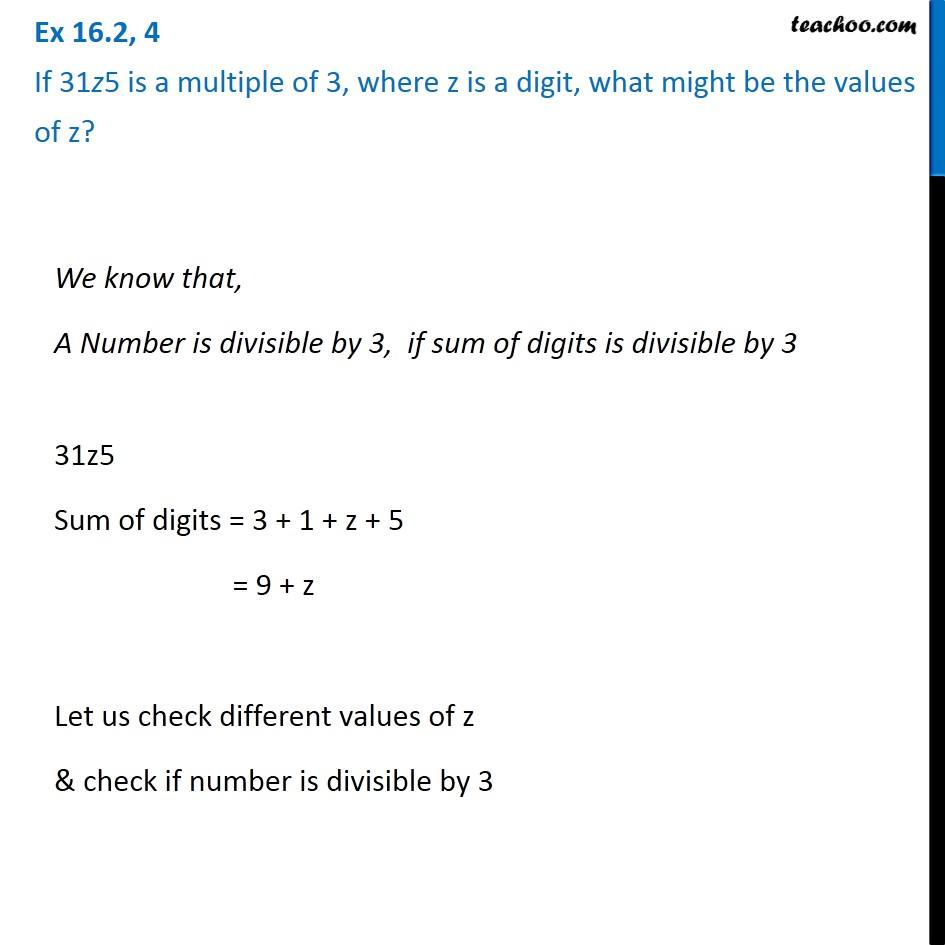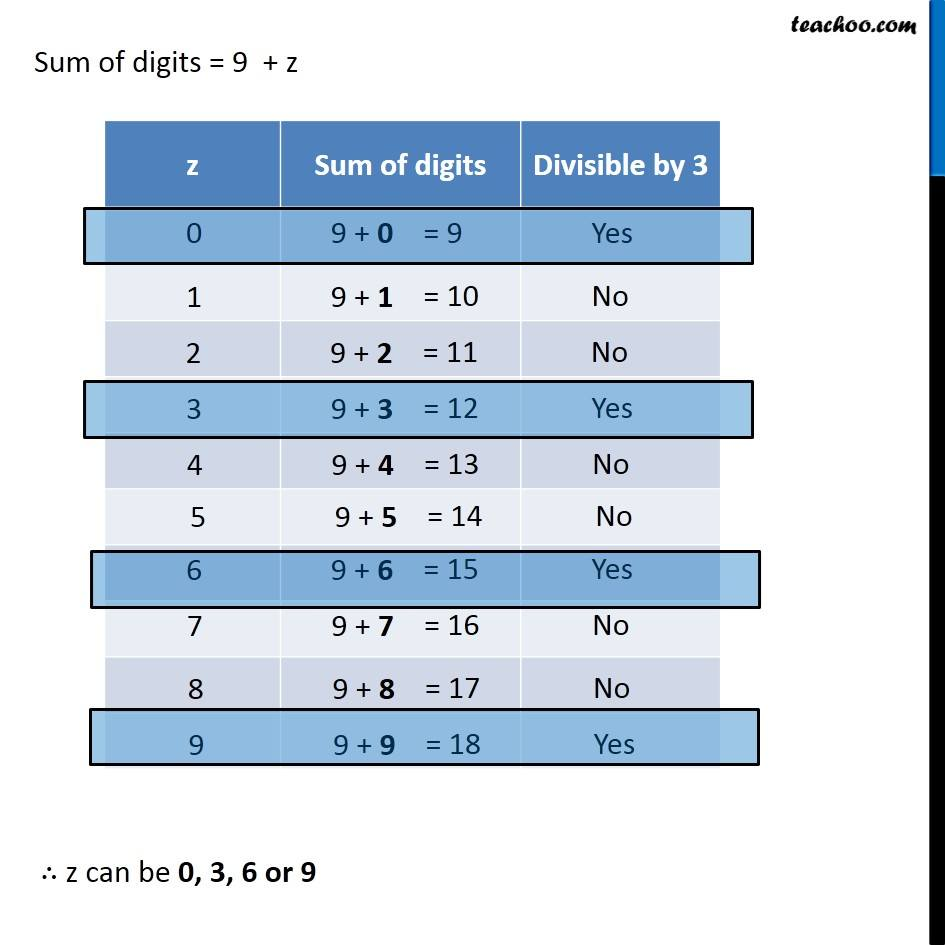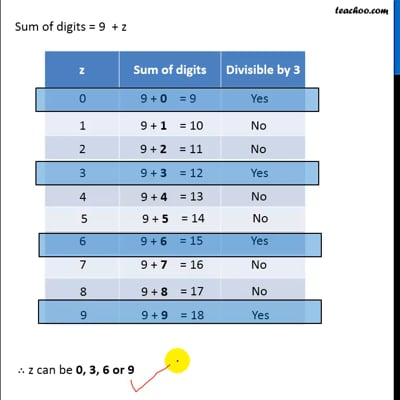Tests of Divisibility

Chapter 16 Class 8 Playing with Numbers
Concept wiseThis video is only available for Teachoo black users

Solve all your doubts with Teachoo Black (new monthly pack available now!)

### Transcript

Ex 16.2, 4 If 31z5 is a multiple of 3, where z is a digit, what might be the values of z? We know that, A Number is divisible by 3, if sum of digits is divisible by 3 31z5 Sum of digits = 3 + 1 + z + 5 = 9 + z Let us check different values of z & check if number is divisible by 3 Sum of digits = 9 + z ∴ z can be 0, 3, 6 or 9